Скачать презентацию Personal Finance Another Perspective Time Value of Money

b0ae5f8aa8fb6dc9cc22f1bcf3ee4595.ppt

• Количество слайдов: 24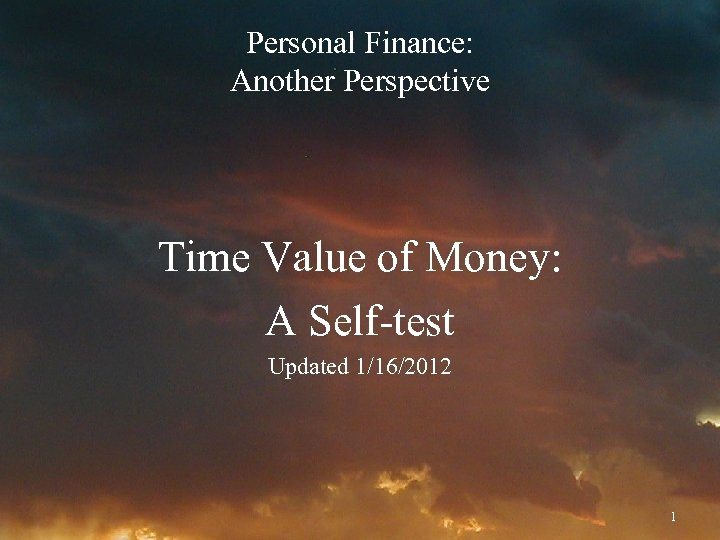Personal Finance: Another Perspective Time Value of Money: A Self-test Updated 1/16/2012 1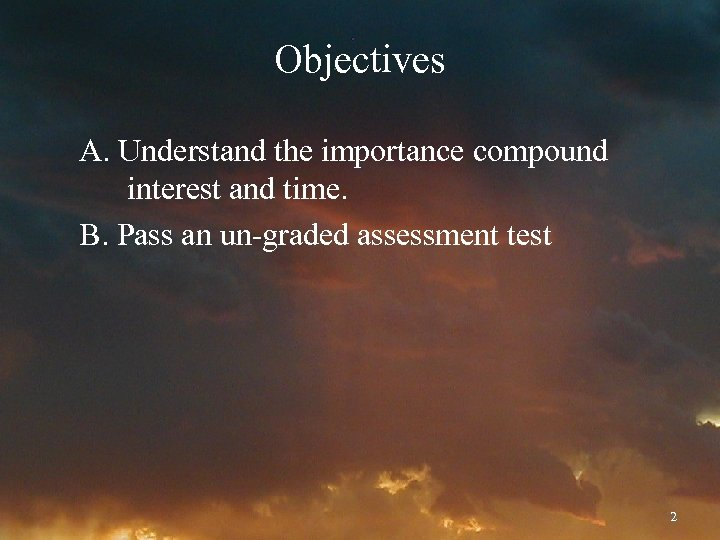Objectives A. Understand the importance compound interest and time. B. Pass an un-graded assessment test 2How Important is Interest? Albert Einstein stated: “Compound interest is the eighth wonder of the world. ” Following are seven “Time Value of Money” problems to test your knowledge. You should already know how to do these types of problems. 3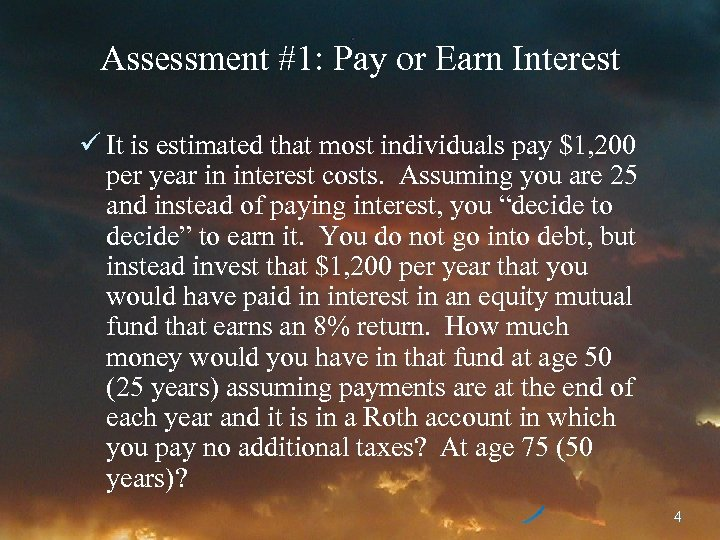Assessment #1: Pay or Earn Interest ü It is estimated that most individuals pay \$1, 200 per year in interest costs. Assuming you are 25 and instead of paying interest, you “decide to decide” to earn it. You do not go into debt, but instead invest that \$1, 200 per year that you would have paid in interest in an equity mutual fund that earns an 8% return. How much money would you have in that fund at age 50 (25 years) assuming payments are at the end of each year and it is in a Roth account in which you pay no additional taxes? At age 75 (50 years)? 4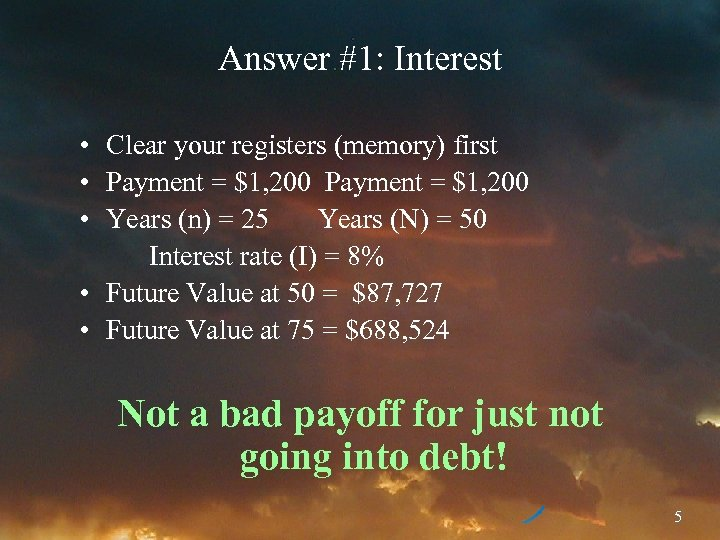Answer #1: Interest • Clear your registers (memory) first • Payment = \$1, 200 • Years (n) = 25 Years (N) = 50 Interest rate (I) = 8% • Future Value at 50 = \$87, 727 • Future Value at 75 = \$688, 524 Not a bad payoff for just not going into debt! 5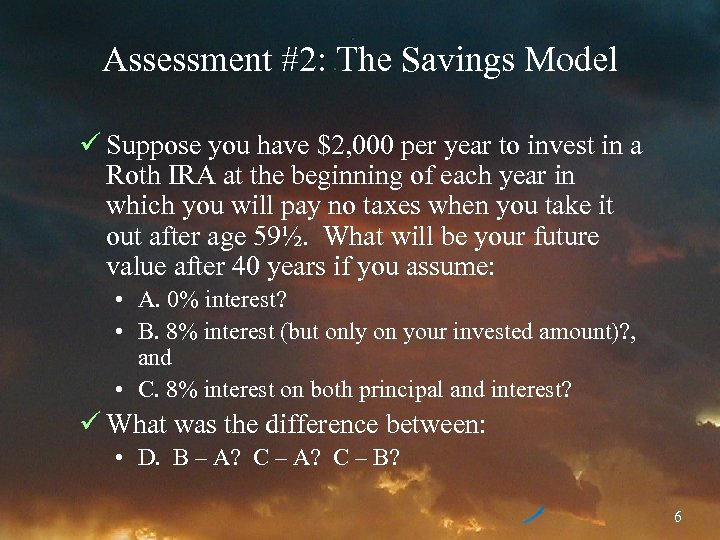Assessment #2: The Savings Model ü Suppose you have \$2, 000 per year to invest in a Roth IRA at the beginning of each year in which you will pay no taxes when you take it out after age 59½. What will be your future value after 40 years if you assume: • A. 0% interest? • B. 8% interest (but only on your invested amount)? , and • C. 8% interest on both principal and interest? ü What was the difference between: • D. B – A? C – B? 6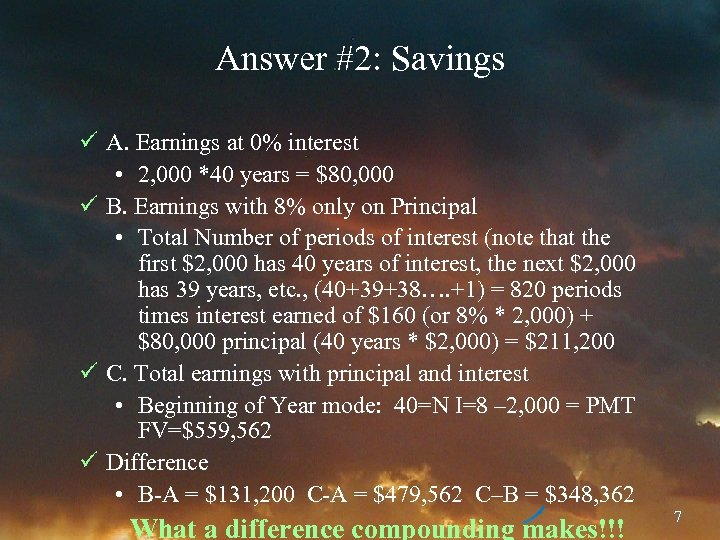Answer #2: Savings ü A. Earnings at 0% interest • 2, 000 *40 years = \$80, 000 ü B. Earnings with 8% only on Principal • Total Number of periods of interest (note that the first \$2, 000 has 40 years of interest, the next \$2, 000 has 39 years, etc. , (40+39+38…. +1) = 820 periods times interest earned of \$160 (or 8% * 2, 000) + \$80, 000 principal (40 years * \$2, 000) = \$211, 200 ü C. Total earnings with principal and interest • Beginning of Year mode: 40=N I=8 – 2, 000 = PMT FV=\$559, 562 ü Difference • B-A = \$131, 200 C-A = \$479, 562 C–B = \$348, 362 What a difference compounding makes!!! 7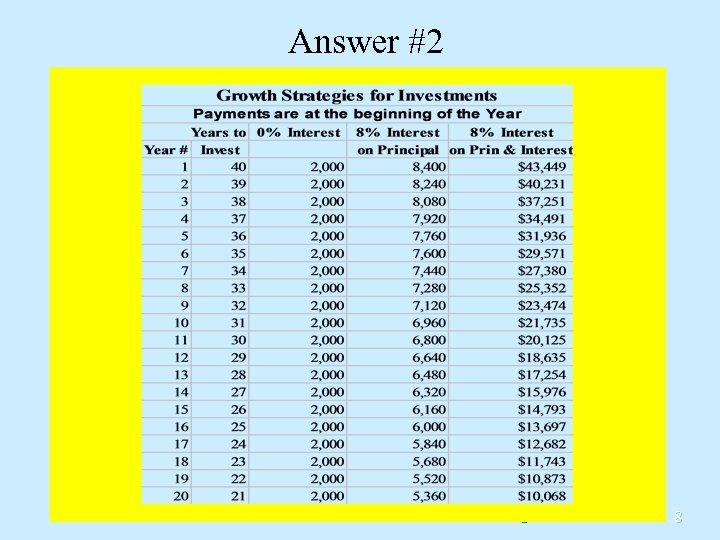Answer #2 8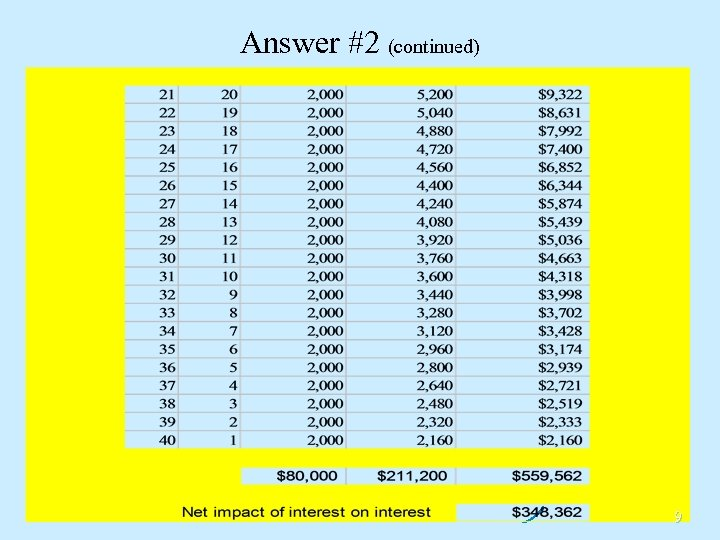Answer #2 (continued) 9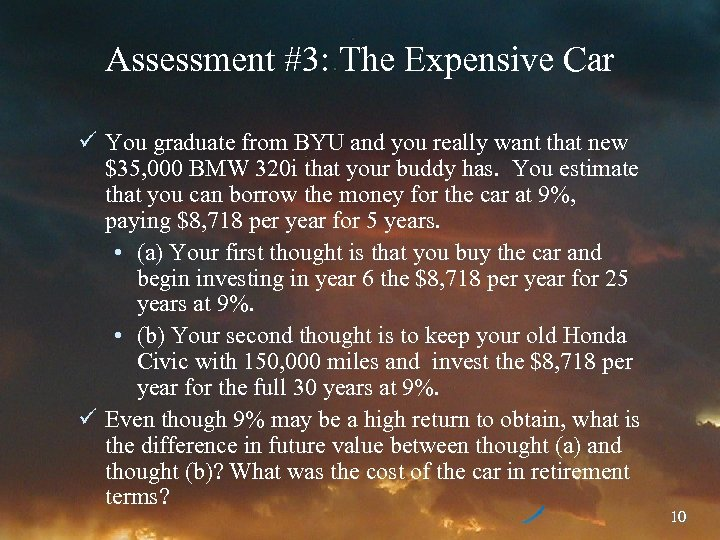Assessment #3: The Expensive Car ü You graduate from BYU and you really want that new \$35, 000 BMW 320 i that your buddy has. You estimate that you can borrow the money for the car at 9%, paying \$8, 718 per year for 5 years. • (a) Your first thought is that you buy the car and begin investing in year 6 the \$8, 718 per year for 25 years at 9%. • (b) Your second thought is to keep your old Honda Civic with 150, 000 miles and invest the \$8, 718 per year for the full 30 years at 9%. ü Even though 9% may be a high return to obtain, what is the difference in future value between thought (a) and thought (b)? What was the cost of the car in retirement terms? 10Answer #3: The Car ü Payment = \$8, 718, N = 25, I = 9% • Future value = \$738, 422 ü Payment = \$8, 718, N = 30, I = 9% • Future value = \$1, 188, 329 ü The cost of the car in retirement terms is \$449, 907 That is one expensive beamer! 11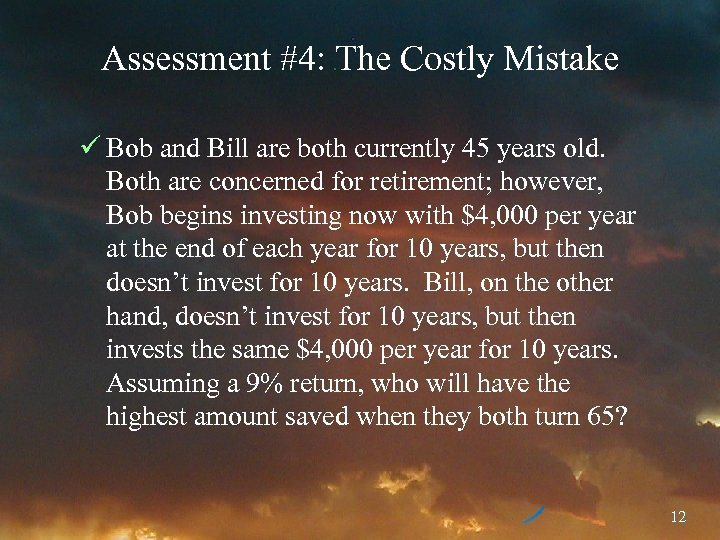Assessment #4: The Costly Mistake ü Bob and Bill are both currently 45 years old. Both are concerned for retirement; however, Bob begins investing now with \$4, 000 per year at the end of each year for 10 years, but then doesn’t invest for 10 years. Bill, on the other hand, doesn’t invest for 10 years, but then invests the same \$4, 000 per year for 10 years. Assuming a 9% return, who will have the highest amount saved when they both turn 65? 12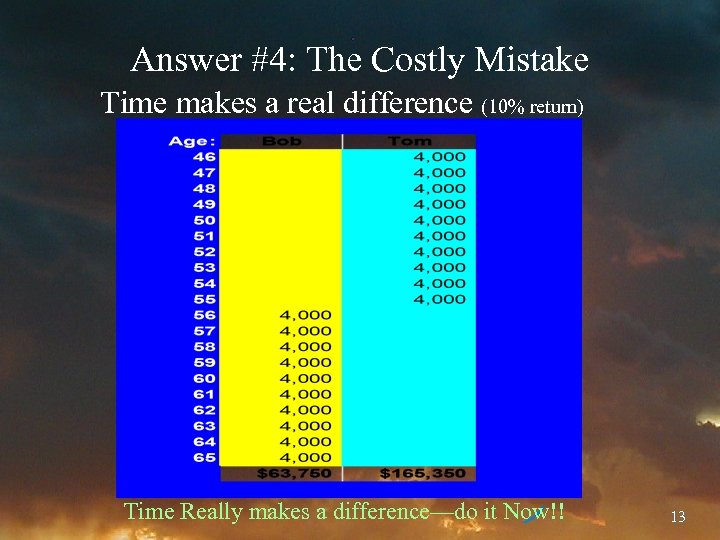Answer #4: The Costly Mistake Time makes a real difference (10% return) Time Really makes a difference—do it Now!! 13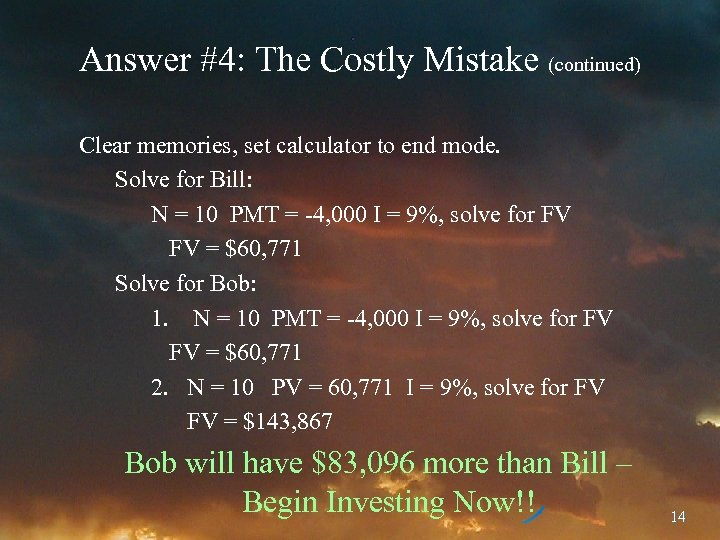Answer #4: The Costly Mistake (continued) Clear memories, set calculator to end mode. Solve for Bill: N = 10 PMT = -4, 000 I = 9%, solve for FV FV = \$60, 771 Solve for Bob: 1. N = 10 PMT = -4, 000 I = 9%, solve for FV FV = \$60, 771 2. N = 10 PV = 60, 771 I = 9%, solve for FV FV = \$143, 867 Bob will have \$83, 096 more than Bill – Begin Investing Now!! 14Assessment #5: Adjusting for Inflation ü Assuming you have an investment making a 30% return, and inflation of 20%, what is your real return on this investment? 15Answer #5: Inflation The traditional (and incorrect) method for calculating real returns is: Nominal return – inflation = real return. This would give: 30% - 20% = 10% The correct method is: (1+nominal return)/(1+inflation) – 1 = real return (1. 30/1. 20)-1 = 8. 33% The traditional method overstates return in this example by 20% (10%/8. 33%) Be very careful of inflation, especially high inflation!! 16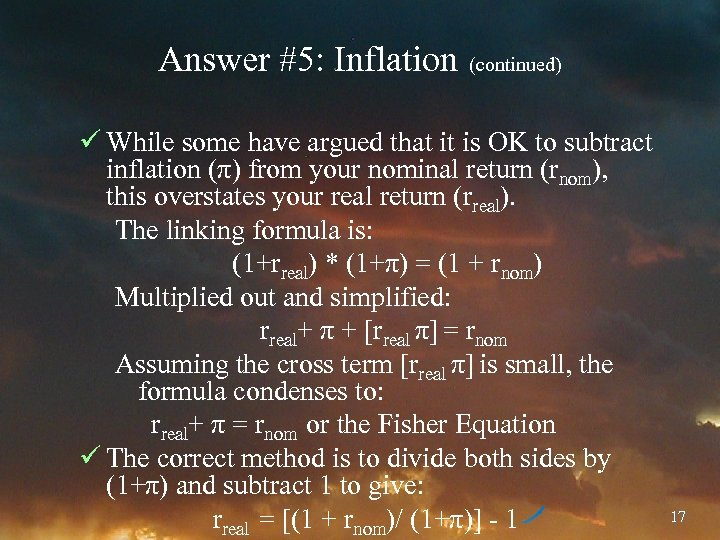Answer #5: Inflation (continued) ü While some have argued that it is OK to subtract inflation (π) from your nominal return (rnom), this overstates your real return (rreal). The linking formula is: (1+rreal) * (1+π) = (1 + rnom) Multiplied out and simplified: rreal+ π + [rreal π] = rnom Assuming the cross term [rreal π] is small, the formula condenses to: rreal+ π = rnom or the Fisher Equation ü The correct method is to divide both sides by (1+π) and subtract 1 to give: rreal = [(1 + rnom)/ (1+π)] - 1 17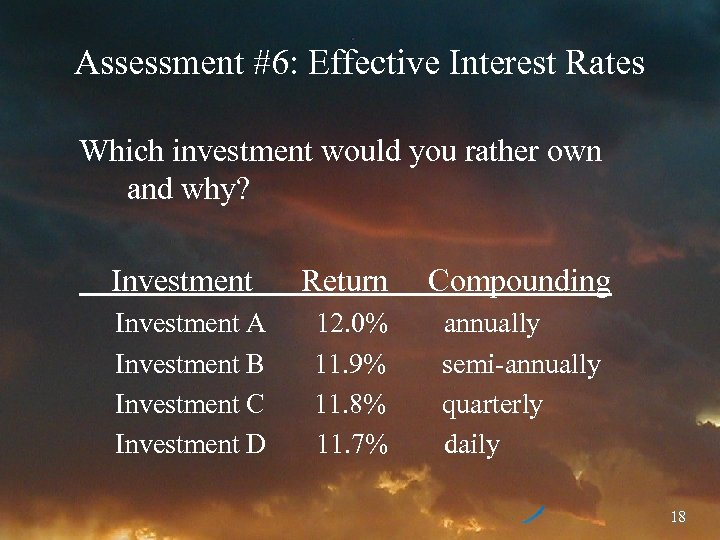Assessment #6: Effective Interest Rates Which investment would you rather own and why? Investment A Investment B Investment C Investment D Return Compounding 12. 0% 11. 9% 11. 8% 11. 7% annually semi-annually quarterly daily 18Answer #6: Effective Interest Rates The formula is ((1 + return/period)^period) – 1 l (1+. 12/1)1 -1 = 12. 00% l (1+. 119/2)2 – 1 = 12. 25% l (1+. 118/4)4 – 1 = 12. 33% l (1+. 117/365)365 – 1 = 12. 41% Even though D has a lower annual return, due to the compounding, it has a higher effective interest rate. How you compound makes a difference! 19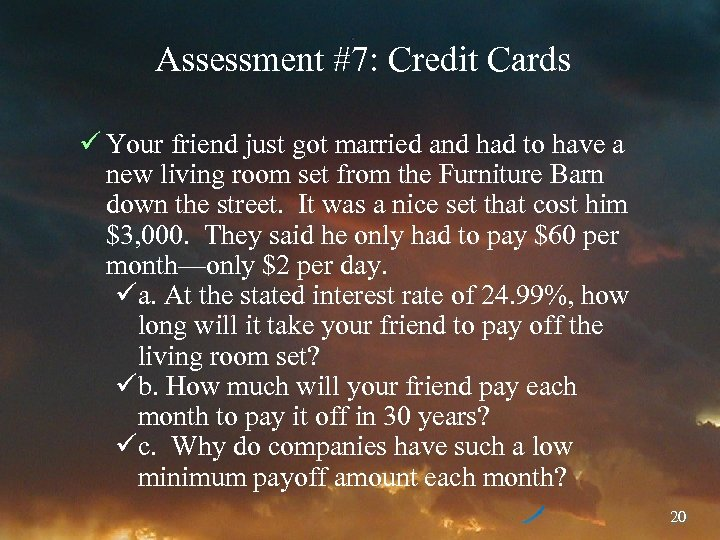Assessment #7: Credit Cards ü Your friend just got married and had to have a new living room set from the Furniture Barn down the street. It was a nice set that cost him \$3, 000. They said he only had to pay \$60 per month—only \$2 per day. üa. At the stated interest rate of 24. 99%, how long will it take your friend to pay off the living room set? üb. How much will your friend pay each month to pay it off in 30 years? üc. Why do companies have such a low minimum payoff amount each month? 20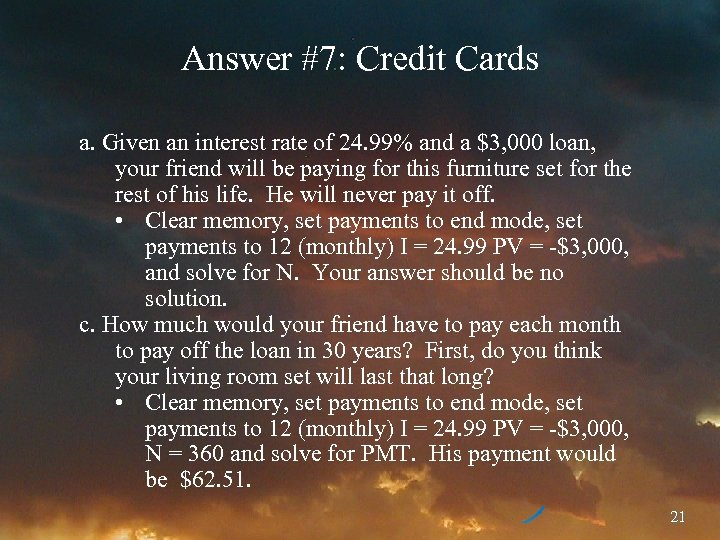Answer #7: Credit Cards a. Given an interest rate of 24. 99% and a \$3, 000 loan, your friend will be paying for this furniture set for the rest of his life. He will never pay it off. • Clear memory, set payments to end mode, set payments to 12 (monthly) I = 24. 99 PV = -\$3, 000, and solve for N. Your answer should be no solution. c. How much would your friend have to pay each month to pay off the loan in 30 years? First, do you think your living room set will last that long? • Clear memory, set payments to end mode, set payments to 12 (monthly) I = 24. 99 PV = -\$3, 000, N = 360 and solve for PMT. His payment would be \$62. 51. 21Answer #7: Credit Cards (continued) C. Why do companies have such a low minimum payoff amount each month? • So they can earn lots of your money from fees and interest! • This is money you shouldn’t be paying them—Earn interest, don’t pay interest! Minimum payments are not to be nice, but to keep you paying them interest! 22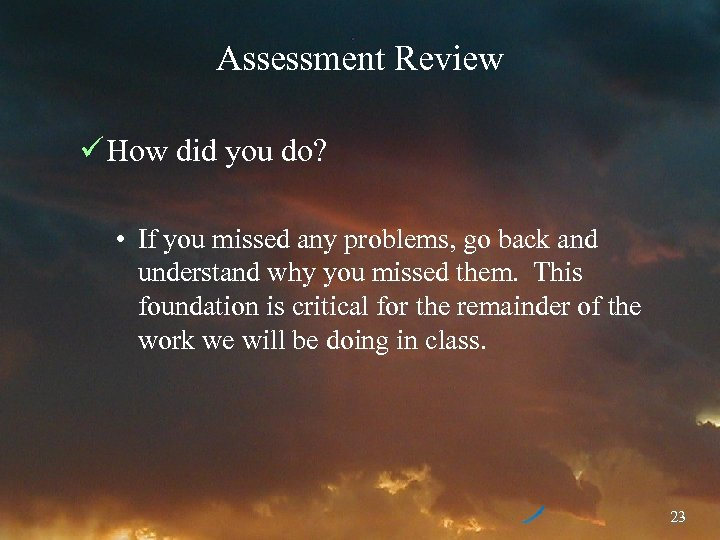Assessment Review ü How did you do? • If you missed any problems, go back and understand why you missed them. This foundation is critical for the remainder of the work we will be doing in class. 23Review of Objectives A. Do you understand the importance compound interest and time? B. Did you pass the un-graded assessment test? 24